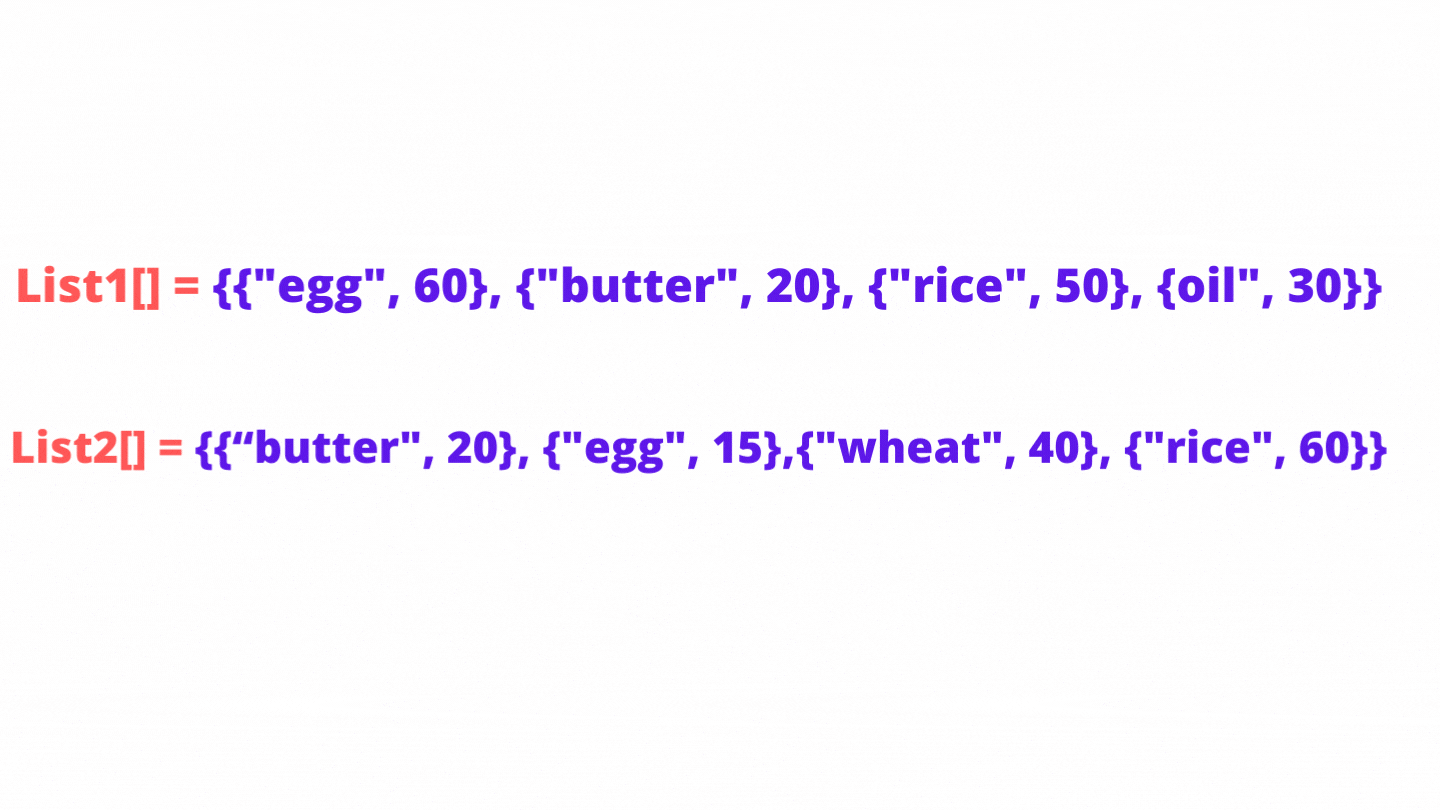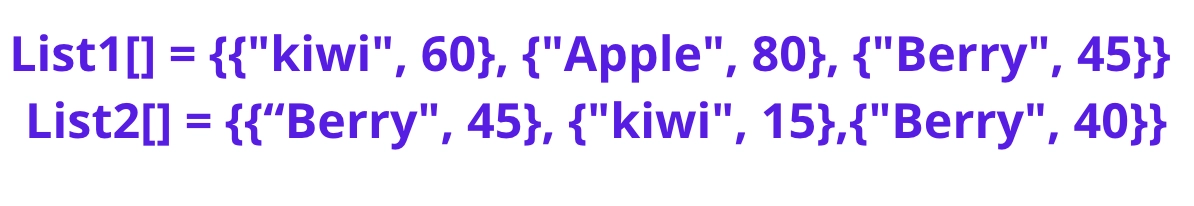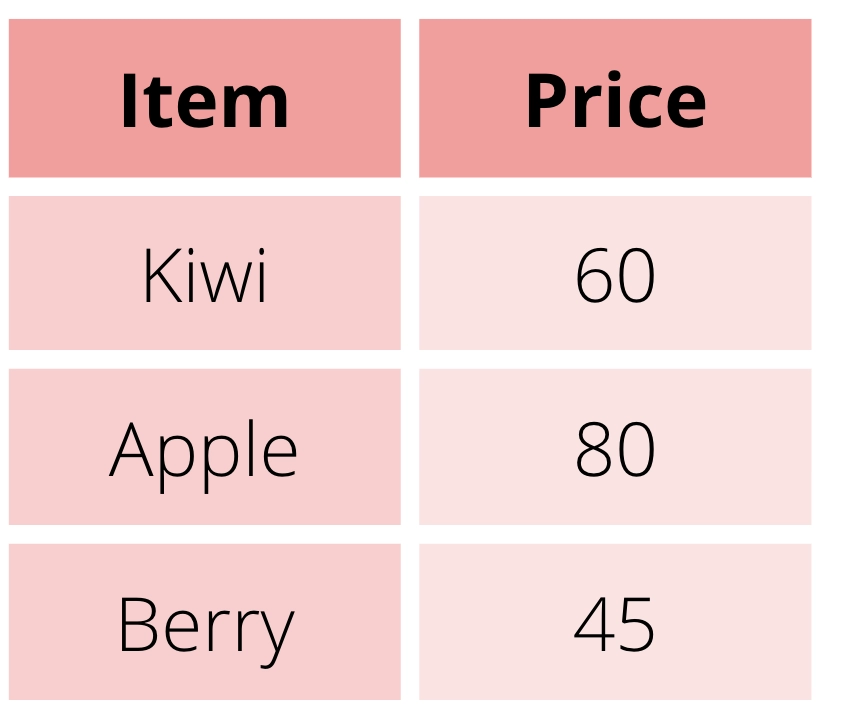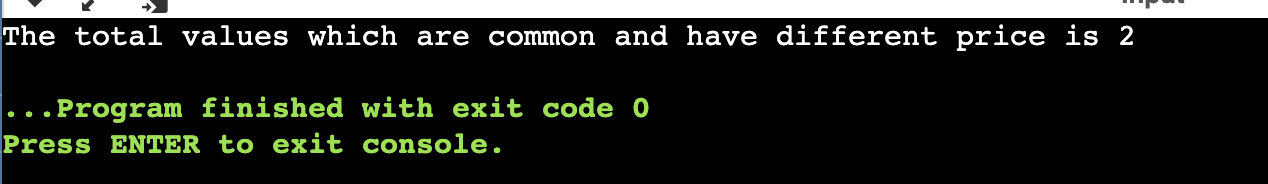New update is available. Click here to update.
Last Updated: Jun 30, 2023
Easy

# Count Items Common to Both the Lists But With Different Prices

## Introduction

In this article, we’ll be looking at one problem named Count items common to both the lists but with different prices using hashing.

​​But, before we start let us revise the concept of hashing. So, What is Hashing?

The most common application of hashing is to implement hash tables. A hash table is a list that stores key/value pairs that can be accessed using their index.

This gives a programmer a great way to retrieve and manipulate the data in an efficient manner.

You’ll be amazed at how hash tables made the work easy for us in just seconds.

So, let us start:### Problem Statement

You are given two lists. Each of these indexes contains the item's name and price. The problem statement instructs you to count items that are common to both lists but have different prices, which is to determine how many items are common to both lists but have different prices.

Input Test Case

OutputExplanationDetailed Explanation

=> Count = 0

• The first common item found is {egg, 60} and {egg, 15}, hence we can consider this.
Count = Count + 1
• The next common item found is {butter, 20} and {butter, 20}, as we can see, it has the same price hence we cannot consider this.
The count remains the same.
• The next common item found is {rice, 50} and {rice, 60}, hence we can consider this.
Count = Count + 1

Let us now discuss how to solve the above problem:

## Approach - Hashing for Count Items Common to Both the Lists But With Different Prices

### Idea

The idea is to use a map and add either of the lists as a key-value pair to the map. After successfully adding the items and prices from list 1 to the map, we can check whether the current element of the second list is present in the map or not. If it is present, its price must be different. If this is the case, increase the count. Otherwise, proceed to the next element.

Let us look at its algorithm for better understanding:

### Algorithm

1. Declare a map and set the output value to 0.

2. Add the name and price of the first list to the map.

3. Go through the second list.

3.1  Look for the element of the second list if each element's name in the second list has a common value in list1's name.

3.2  Determine whether the price of that specific element should differ from the price of the element in list1.

4. If true, increase the output count by one.

5. Return the output.

### Pictorial Representation

Step 1: Take two ListsStep 2: Add first list into map.Step 3: Traverse through the second list and check whether the current item is present in the map with a different price or not and increment the count accordingly.

Output will be:Now, let us use our hands to implement the same:

## Implementation in C++

``````/*
* C++ Program to Count items common to both the lists but with different prices
*/
#include<bits/stdc++.h>
using namespace std;

/*
* item will have name and price
*/
struct item
{
string name;
int price;
};
/*
* Utility function to return the count
*/
int getItemCount(item list1[], int m, item list2[], int n)
{
// Initially the count is 0
int count = 0;
// Map for storing the first list
unordered_map<string, int> MAP;

// Storing the values of list1 into map
for (int i = 0; i < m; i++)
MAP[list1[i].name] = list1[i].price;
// Traversing over the second list
for (int i = 0; i < n; i++)
if ((MAP.find(list2[i].name) != MAP.end()) &&(MAP[list2[i].name] != list2[i].price))
count++;
return count;
}
/*
* main function
*/
int main()
{
/*
* define the list 1 and list 2
*/
item list1[] = {{"egg", 60}, {"butter", 20}, {"rice", 50}, {"oil", 30}};
item list2[] = {{"butter", 20}, {"egg", 15}, {"wheat", 40}, {"rice", 60}};
int m = sizeof(list1) / sizeof(list1);
int n = sizeof(list2) / sizeof(list2);
/*
* Calling getItemCount and printing the result
*/
cout<< "The total values which are common and have different price is " <<getItemCount(list1, m, list2, n);
return 0;
}``````

Output## Implementation in Java

``````/*
Java program to count items common to both the lists but with different prices
*/
import java.util.*;
class CountItems
{
/*
* item will have name and price
*/
public static class item
{
String name;
int price;
item(String name, int price)
{
this.name=name;
this.price=price;
}
};
/*
* Utility function to return the output
*/
public static int getItemCount(item list1[], int m,item list2[], int n)
{
// Set the count as 0
int count = 0;
// Hashmap to store the list 1 into hashmap
HashMap<String, Integer> MAP=new HashMap<>();
// Add the values of list 1 into map
for (int i = 0; i < m; i++)
{
MAP.put(list1[i].name, list1[i].price);
}
// Traverse through the second list
for (int i = 0; i < n; i++)
{
if ((MAP.containsKey(list2[i].name) && (MAP.get(list2[i].name) != list2[i].price)))
count++;
}
// finally return the count
return count;
}
public static void main(String [] args)
{
item list1[] = {new item("egg", 60), new item("butter", 20),new item("rice", 50), new item("oil", 30)};
item list2[] = {new item("butter", 20), new item("egg", 15),new item("wheat", 40), new item("rice", 60)};
int m = list1.length;
int n = list2.length;
System.out.println("The total values which are common and have different price is " + getItemCount(list1, m, list2, n));
}
}``````

Output## Complexity Analysis

Time Complexity: O(m+n) where “m” and “n” is the number of elements in list1 and list2.

Space Complexity: Since we are storing the elements only of list 1 in the map, the space complexity is dependent only on the size of list1. Thus space complexity is O(m) where “m” is the number of elements in the list.

Check out this problem - Pair Sum In Array.

### What is a hashmap in java?

The Java collections framework's HashMap class implements the functionality of the hash table data structure. It organises elements into key/value pairs. In this context, keys are unique identifiers that are used to associate each value on a map. The Map interface is implemented by the HashMap class.

### Where do we use a hashset?

For high-performance operations involving a set of unique data, a hashset is typically utilised. HashSet's internal structure is enhanced for quicker searches because it only contains unique components.

### What is the HashMap searching time complexity?

For lookup, HashMap takes O(1) complexity.

### What is the HashMap insertion time complexity?

For insertion, HashMap takes O(1) complexity.

### What is the difference between ordered and unordered_map in C++?

The main difference is Ordering. The items in the ordered map are stored in increasing order whereas in the unordered_map there is no ordering.

## Conclusion

To wrap up the article, we’ve discussed the approach and implementation for Count items common to both the lists but with different prices in different languages.

Check out here for similar problem statement blogs:

Refer to our guided paths on Coding Ninjas Studio to learn more about DSA, JavaScript, System Design, DBMS, Java, etc. Enrol in our courses and refer to the mock test and problems available. Take a look at the interview experiences and interview bundle for placement preparations.

Do upvote our blog to help other ninjas grow. 🥷✨Happy Learning, Ninja!